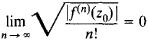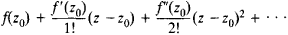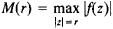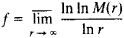# Entire Function

Also found in: Wikipedia.

## entire function

[en¦tīr ¦fəŋk·shən]
(mathematics)
A function of a complex variable which is analytic throughout the entire complex plane. Also known as integral function.
McGraw-Hill Dictionary of Scientific & Technical Terms, 6E, Copyright © 2003 by The McGraw-Hill Companies, Inc.
The following article is from The Great Soviet Encyclopedia (1979). It might be outdated or ideologically biased.

## Entire Function

(or integral function), a function of a complex variable that is analytic throughout the entire complex plane. Examples of entire functions are the algebraic polynomial a0 + a1z + · · · + anzn and the functions sin z, cos z, and ez.

The point at infinity is in general an isolated singularity of an entire function. In order for the point at infinity to be a removable singularity of the entire function f(z), it is necessary and sufficient that f(z) be a constant. The point at infinity is a pole of f(z) if, and only if, f(z) is an algebraic polynomial. If the point z = ∞ is an essential singularity of f(z), then f(z) is said to be a transcendental entire function. Examples are the functions sin z, cos z, and ez.

In order for f(z) to be an entire function, it is necessary and sufficient that the relationhold for at least one point z0. In this case the expansion of f(z) in the Taylor’s seriesconverges throughout the entire complex plane.

The classification of transcendental entire functions is based on the rate of increase M(r) of the function; M(r) is defined by the equationThe quantityis called the order of the entire function f(z). The relation between the order of an entire function and the distribution of its zeros was established by H. Poincaré, J. Hadamard, and E. Borel.

### REFERENCE

Markushevich, A. I. Tselye funktsii. Moscow, 1965.
References in periodicals archive ?
If b = 0, then [zeta](s, [alpha]; b) is an entire function.